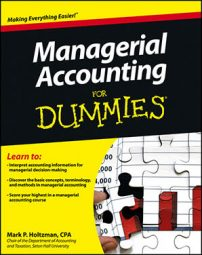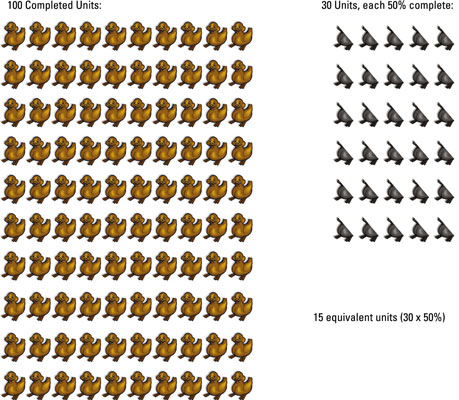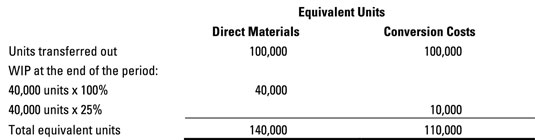##### Managerial Accounting For DummiesThe second part of the cost of production report accounts for the units that the first part indicates the department is responsible for. During April, the Balloon department finished working on 1,900 clowns, sending them to the next department. At the end of April, the Balloon folks still had 800 clowns of WIP on the assembly line, waiting for their balloons.

This progress means that the Balloon department has accounted for 2,700 units:

Units accounted for = Units completed + Ending work-in-process units = 1,900 + 800 = 2,700

The number of units to account for must equal the number of units accounted for. This is a factory, not a circus.

To measure how much work each WIP department did, accountants use the term equivalent units. Equivalent units sum up the fractions of units actually completed. For example, if a department got halfway through two separate units, it would count that work as one equivalent unit.

What’s the difference? Suppose an assembly line has 24 toys that are 50 percent complete. However, 24 toys that are only 50 percent complete comprise 12 equivalent units because that measure represents the amount of work that each WIP department actually did. They got 50 percent of the way through 24 units, which comes out to 12 equivalent units.

To compute the number of equivalent units of production, just add the total number of units completed and transferred out to the number of equivalent units of ending WIP:

Equivalent units of production = Units completed + (Units in ending work-in-process x Percentage complete)

For example, suppose that the Theresa Toy Factory Assembly department made and finished 100 rubber ducks. It also had 30 units still in process left over at the end of the period, all half complete. These unfinished ducks equal 15 equivalent units (30 units x 0.5 complete). The total equivalent units of production equal 115:

Equivalent units of production = Units completed + (Units in ending work-in-process x Percentage complete)
= 100 + (30 x 50%)
= 115You can also apply the equivalent units concept to direct labor and overhead costs. These costs are sometimes referred to as conversion costs because they’re the costs of converting direct materials into finished goods.

The degree of completion with respect to direct materials may differ from conversion costs because direct materials usually get added at the beginning of the production process, whereas conversion costs get added throughout the production process.

For example, maybe your inventory is 100 percent complete with respect to direct materials (because all the materials have been added) but only, say, 10 percent complete with respect to conversion costs. Therefore, the same inventory may actually have two different equivalent units of production: one for direct materials and another for conversion costs.

Now think about another example. The Turbo Paper Clip Company’s Bending department bends paper clips into their proper shape. The Bending department transferred 100,000 completely bent paper clips into the Boxing department. At the end of the period, Bending had 40,000 units of WIP on hand that were 100 percent complete with respect to direct materials, but only 25 percent complete with respect to conversion costs.

To compute the equivalent units produced by the Bending department, apply the same formula as you did for the ducks, but do so twice: once for direct materials and a second time for conversion costs.